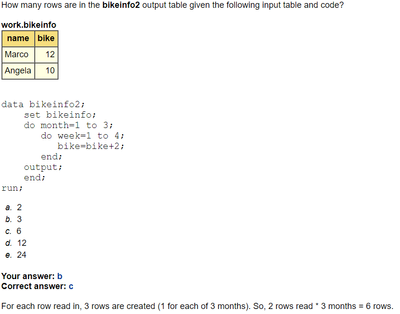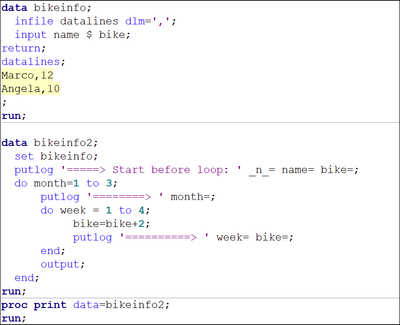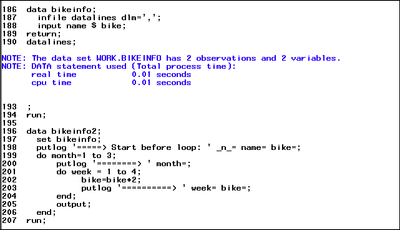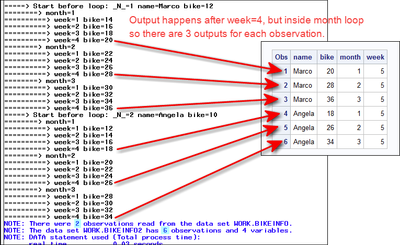## Question on Do loop

Hi All,

Can anybody elaborate the explanation for the below question please? I believe only one row is read for each month when it's an implicit output.1 ACCEPTED SOLUTION

Accepted Solutions

## Re: Question on Do loop

Hi:

Where you are in doubt about the answer to the quizzes in the class, it's always a good idea to write a program to test the program logic so you can understand exactly what's happening. This is where using the class techniques like PUTLOG will help you. For example, you can write a simple program to make the input bikeinfo file. Then the DATA step is given in the quiz question:The program runs successfully and in the LOG, you see:Then you can compare the PUTLOG statements in the LOG to the output from PROC PRINT:And the log shows you that 2 observations were read from WORK.BIKEINFO but that 6 observations were written to WORK.BIKEINFO2. If you compare the PUTLOG output to the PROC PRINT, you can see exactly which 6 observations were written out, one for each value of MONTH. You can tell the output was OUTSIDE of the DO loop for WEEK because the value of WEEK is 5, which means that the loop for week had ended and that the OUTPUT happened after week had been incremented from 4 to 5, which ended the WEEK loop.

Hope this helps explain a possible approach to validating the quiz answers in the classes.

Cynthia

3 REPLIES 3

## Re: Question on Do loop

The question is not how many values are calculated, but how many OUTPUTs are implemented.

--------------------------
The hash OUTPUT method will overwrite a SAS data set, but not append. That can be costly. Consider voting for Add a HASH object method which would append a hash object to an existing SAS data set

Would enabling PROC SORT to simultaneously output multiple datasets be useful? Then vote for
Allow PROC SORT to output multiple datasets

--------------------------

## Re: Question on Do loop

There are two input lines.
There is a loop that runs for each line and outputs 3 times. So 2*3 =6.

This uses an explicit output not implicit.

@Neeharika89 wrote:

Hi All,

Can anybody elaborate the explanation for the below question please? I believe only one row is read for each month when it's an implicit output.## Re: Question on Do loop

Hi:

Where you are in doubt about the answer to the quizzes in the class, it's always a good idea to write a program to test the program logic so you can understand exactly what's happening. This is where using the class techniques like PUTLOG will help you. For example, you can write a simple program to make the input bikeinfo file. Then the DATA step is given in the quiz question:The program runs successfully and in the LOG, you see:Then you can compare the PUTLOG statements in the LOG to the output from PROC PRINT:And the log shows you that 2 observations were read from WORK.BIKEINFO but that 6 observations were written to WORK.BIKEINFO2. If you compare the PUTLOG output to the PROC PRINT, you can see exactly which 6 observations were written out, one for each value of MONTH. You can tell the output was OUTSIDE of the DO loop for WEEK because the value of WEEK is 5, which means that the loop for week had ended and that the OUTPUT happened after week had been incremented from 4 to 5, which ended the WEEK loop.

Hope this helps explain a possible approach to validating the quiz answers in the classes.

Cynthia

Discussion stats
• 3 replies
• 598 views
• 4 likes
• 4 in conversation